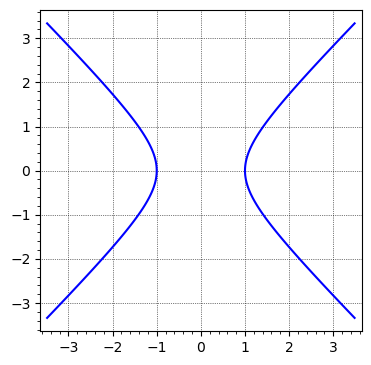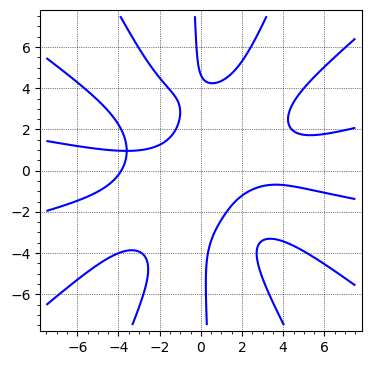# Select component of an implicit_plot curve

I'd like to plot one of the connected components of a plot. This is like

sage: x, y = SR.var('x, y', domain='real')
sage: F0 = x^2 - y^2 - 1
sage: B0 = 3.5
sage: G0 = implicit_plot(F0, (x, -B0, B0), (y, -B0, B0), gridlines=True)
sage: G0.show(figsize=5)
Launched png viewer for Graphics object consisting of 1 graphics primitivewhich one could solve and manually select the connected components. In my case, I deal with an equation that is inconvenient to plot directly from the solution. Is there a command or a feature to implicit plot' which has the effect of "taking this connected component of the solution" (e.g. in the example, passing through $(-1,0)$?) Also I wouldn't like to restrict to either positive or negative $x$ (my curve is somehow more complicated and restricting to regions doesn't work).

I excluded the curve to make this a minimal example, but it's probably helpful to know that I don't know how to easily parametrize it. Is something like

   sage: F = -1/720*x^6*y*cos(sqrt(2)*pi) + 1/144*x^4*y^3*cos(sqrt(2)*pi) - 1/240*x^2*y^5*cos(sqrt(2)*pi)+ 1/5040*y^7*cos(sqrt(2)*pi) - 1/5040*x^7*sin(sqrt(2)*pi) + 1/240*x^5*y^2*sin(sqrt(2)*pi) -1/144*x^3*y^4*sin(sqrt(2)*pi) + 1/720*x*y^6*sin(sqrt(2)*pi) +1/114688*720^(2/5)*abs(-2*sqrt(5) + 10)^(7/2)*cos(2/5*sqrt(2)*pi)*cos(7/2*arctan2(0, -2*sqrt(5) + 10))   + 1/114688*720^(2/5)*abs(-2*sqrt(5) + 10)^(7/2)*sin(2/5*sqrt(2)*pi)*sin(7/2*arctan2(0, -2*sqrt(5) + 10))- 3/8192*720^(2/5)*sqrt(5)*abs(-2*sqrt(5) + 10)^(5/2)*cos(2/5*sqrt(2)*pi)*cos(5/2*arctan2(0,  -2*sqrt(5) + 10)) - 3/8192*720^(2/5)*sqrt(5)*abs(-2*sqrt(5) + 10)^(5/2)*sin(2/5*sqrt(2)*pi)   *sin(5/2*arctan2(0, -2*sqrt(5) + 10)) - 9/8192*720^(2/5)*abs(-2*sqrt(5) + 10)^(5/2)   *cos(2/5*sqrt(2)*pi)*cos(5/2*arctan2(0, -2*sqrt(5) + 10)) - 9/8192*720^(2/5)*abs(-2*sqrt(5)  + 10)^(5/2)*sin(2/5*sqrt(2)*pi)*sin(5/2*arctan2(0, -2*sqrt(5) + 10))+ 15/2048*720^(2/5)*sqrt(5)*abs(-2*sqrt(5) + 10)^(3/2)*cos(2/5*sqrt(2)*pi)*cos(3/2*arctan2(0,   -2*sqrt(5) + 10)) + 15/2048*720^(2/5)*sqrt(5)*abs(-2*sqrt(5)  + 10)^(3/2)*sin(2/5*sqrt(2)*pi)*sin(3/2*arctan2(0, -2*sqrt(5) + 10))  + 35/2048*720^(2/5)*abs(-2*sqrt(5) + 10)^(3/2)*cos(2/5*sqrt(2)*pi)*cos(3/2*arctan2(0, -2*sqrt(5) + 10)) + 35/2048*720^(2/5)*abs(-2*sqrt(5) + 10)^(3/2)*sin(2/5*sqrt(2)*pi)*sin(3/2*arctan2(0, -2*sqrt(5)  + 10)) - 5/64*720^(2/5)*sqrt(5)*sqrt(abs(-2*sqrt(5)  + 10))*cos(2/5*sqrt(2)*pi)*cos(1/2*arctan2(0, -2*sqrt(5) + 10))  - 5/64*720^(2/5)*sqrt(5)*sqrt(abs(-2*sqrt(5) + 10))*sin(2/5*sqrt(2)*pi)*sin(1/2*arctan2(0, -2*sqrt(5) + 10)) - 25/256*720^(2/5)*sqrt(abs(-2*sqrt(5) + 10))*cos(2/5*sqrt(2)*pi)*cos(1/2*arctan2(0, -2*sqrt(5)  + 10)) - 25/256*720^(2/5)*sqrt(abs(-2*sqrt(5) + 10))*sin(2/5*sqrt(2)*pi)*sin(1/2*arctan2(0, -2*sqrt(5)  + 10)) + x*y + 5/56*720^(2/5)*sqrt(5)*sin(2/5*sqrt(2)*pi) - 5/56*720^(2/5)*sin(2/5*sqrt(2)*pi)
sage: B = 7.5
sage: G = implicit_plot(F, (x, -B, B), (y, -B, B), gridlines=True)
sage: G.show(figsize=5)This has many connected components (on the finite plane), the square root of two is just to match another conventions, you could ignore this and any number-theoretical stuff, just think about the sort of not low degree polynomial in two variables. Cannot upload the plot yet.

edit retag close merge delete

After staring at the complicated equation for some time, here is a more concise rephrasing of it.

Putting $z = x + i \, y$, there are some real constants $A$, $B$, $C$ such that the equation amounts to: $$\operatorname{Re}\left(e^{i \, A} \frac{z^7}{7!} + e^{i \, B} \frac{z^2}{2!}\right) = C$$

The degree 7 explains the 7 components of the curve.

The approach in @FrédéricC's answer to Ask Sage question 54765 might give inspiration here.

@slelievre Hi, thanks for the insight and suggestions (and the edit!)

Sort by » oldest newest most voted

Ideally, find a parametric description. Then use a parametric plot with the appropriate range for the parameter.

sage: x, y = SR.va('x, y')
sage: a = acosh(2.)
sage: opt = dict(xmin=-2, xmax=2, ymin=-2, ymax=2, axes=False, frame=True)
sage: Gi = implicit_plot(x^2 - y^2 - 1, (x, -2, 2), (y, -2, 2),
....:                    linewidth=5, color='steelblue', **opt)
sage: Gp = parametric_plot((cosh, sinh), (-a, a),
....:                      thickness=2, color='firebrick', **opt)
sage: Gip = Gi + Gp
sage: P = point2d([(-2, -2), (2, 2)], color='white', alpha=0)
sage: G = graphics_array([P + Gi, P + Gp, P + Gip], ncols=1)
sage: G.show(figsize=12)
`
more

1

thank you for answering. I just don't find a parametrization of my curve (now added to the question's body).